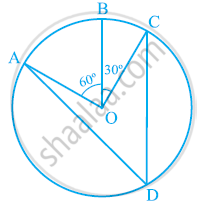# In the given figure, A, B and C are three points on a circle with centre O such that ∠BOC = 30° and ∠AOB = 60°. If D is a point on the circle other than the arc ABC, find ∠ADC. - Mathematics

In the given figure, A, B and C are three points on a circle with centre O such that ∠BOC = 30° and ∠AOB = 60°. If D is a point on the circle other than the arc ABC, find ∠ADC.#### Solution

It can be observed that

∠AOC = ∠AOB + ∠BOC

= 60° + 30°

= 90°

We know that angle subtended by an arc at the centre is double the angle subtended by it any point on the remaining part of the circle.

angleADC=1/2angleAOC=1/2xx90^@ = 45^@

Is there an error in this question or solution?

#### APPEARS IN

NCERT Class 9 Maths
Chapter 10 Circles
Exercise 10.5 | Q 1 | Page 184